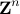The technique of semidefinite programming (SDP) relaxation can be used to obtain a nontrivial bound on the optimal value of a nonconvex quadratically constrained quadratic program (QCQP). We explore concave quadratic inequalities that hold for any vector in the integer lattice, and show that adding these inequalities to a mixed-integer nonconvex QCQP can improve the SDP-based bound on the optimal value. This scheme is tested using several numerical problem instances of the max-cut problem and the integer least squares problem.